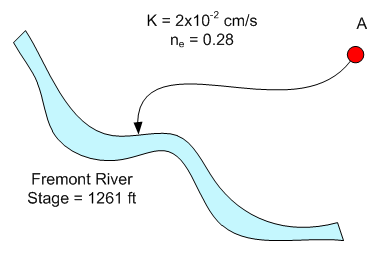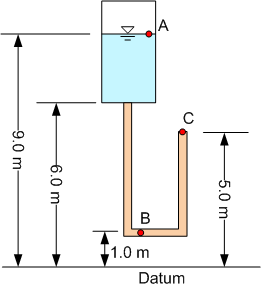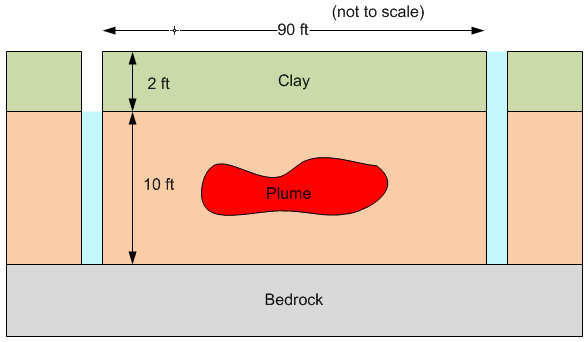# HW#2 - Darcy's Law

1. A flow path computed from a particle tracking analysis is shown below. Point A represents a potential spill location. Water travels from the point A to the river along the path shown. The length of the flow path is approximately 500 ft. The aquifer has a relatively uniform thickness. The water table elevation of point A is 1284 ft. Estimate the travel time (in days) from point A to the river.2. The figure below shows a pressurized reservoir with the water level at point A.  The discharge is into the atmosphere at point C.  The connecting pipe is of constant diameter with a cross-section of 0.02 m^2 and is filled with a sand with hydraulic conductivity of 0.015 m/s.  The average length of the pipe is 9.0 m.  Point B is exactly halfway along the length of the pipe.  The discharge at C is 0.0001 m3/s.  Compute the following:

(a) The head loss from A to C.
(b) The total head at A.
(c) The pressure head at A.
(d) The total head at B.
(e) The pressure head at B
(f) Is there flow from a region of low pressure to a region of high pressure? Where and why?
(g) What would happen to the heads and discharge if the hydraulic conductivity of the medium decreased to 0.001m/s assuming that the water levels and pressures remain constant?3. At a site owned by Company X, it is decided to remediate a plume using a double trench system as shown below. A plume lies between the two trenches in a sand layer that is capped by a relatively impermeable clay layer. Nutrient- and oxygen-laden water will be injected in the trench on the right. The water will flush from right to left and be pumped out of the left trench. To ensure maximum biodegradation, it is best if the sand layer stays saturated. Thus, the trench on the left will be pumped just enough to keep the sand layer saturated. The trench on the right will be kept as full as possible. For the sand layer, assume than n = 0.35 and k = 0.01 cm/s.a) For a one-ft thick slice of the sand layer, how much water would need to be pumped to flush through one pore volume?
b) How long would it take (in days) to pump five pore volumes of water through the sand?